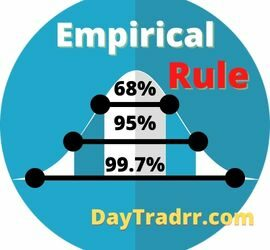# Financial Analysis

68 posts## PEG Ratio Definition – Explanation – Example

PEG Ratio – What Is the Price/Earnings-to-Growth? The PEG ratio is a metric that assists in determining the value of a stock by comparing market price, earnings, and future growth potential. The PEG ratio is the price/earnings to growth ratio.  It is a stock’s price-to-earnings (P/E) ratio divided by the […]## Statement of Retained Earnings: Definition – Explanation – Example

What Is a Statement of Retained Earnings? A statement of retained earnings shows the accumulated portion of a business’s profits that are not distributed as dividends to shareholders. The retained earnings statement is a financial disclosure that shows how a company’s retained earnings have changed over time. Analysts use this […]## Autocorrelation in Statistics and Finance: Overview & Examples

What Is Autocorrelation? Autocorrelation is a statistical representation of the degree of correlation between the same variables measured at successive time intervals. Correlation indicates the strength of a linear relationship between two variables.  Whereas, autocorrelation measures the strength of a linear link between time series lagged values. It measures the […]## Empirical Rule Statistics Definition – Explanation – Example

What Is the Empirical Rule? The Empirical Rule states that virtually all observed data for a normal distribution will fall within three standard deviations of the mean or average. The empirical rule is also known as the three-sigma rule or the 68-95-99.7 rule.  It is a statistical axiom stating that […]## Acid Test Ratio: Explanation – Calculation – Example

What is the Acid Test Ratio? The acid test ratio is the most stringent calculation of short-term liquidity.  It compares a company’s quick assets to its current liabilities. For that reason, the acid-test ratio is also known as the quick ratio. It gauges a company’s liquidity by evaluating how well […]## The Rule of 70 – What Does It Tell An Investor?

What is the Rule of 70? The rule of 70 is a simple method used to estimate how long it will take a person’s money or investment to double depending on the rate of return. This method is widely used for comparing investments with different compounded annual interest rates. While […]